If there are two links,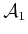and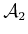, then the C-space can be nicely visualized as a square with opposite faces identified. Each coordinate,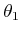and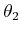, ranges from 0 to, for which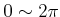. Suppose that each link has length. This yields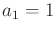. A point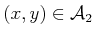is transformed as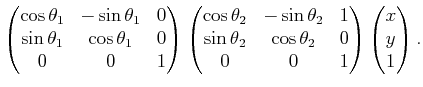(4.58)

To obtain polynomials, the technique from Section 4.2.2 is applied to replace the trigonometric functions usingand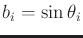, subject to the constraint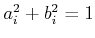. This results in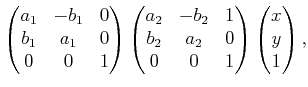(4.59)

for which the constraintsfor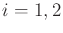must be satisfied. This preserves the torus topology of, but now the C-space is embedded in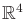. The coordinates of each point are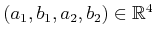; however, there are only two degrees of freedom because each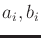pair must lie on a unit circle.

Multiplying the matrices in (4.59) yields the polynomials,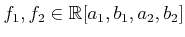,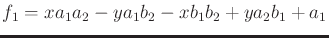(4.60)

and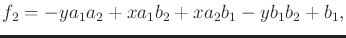(4.61)

for theand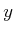coordinates, respectively. Note that the polynomial variables are configuration parameters;andare not polynomial variables. For a given point, all coefficients are determined.

Steven M LaValle 2020-08-14